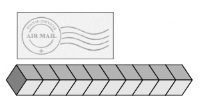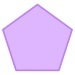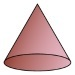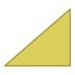##### Notes

Daily Math Practice provides a week's worth of problems ideal for morning work or review. This printable reviews the Grade 1 Common Core Mathematics Standards.

##### Print Instructions

NOTE: Only your test content will print.
To preview this test, click on the File menu and select Print Preview.

See our guide on How To Change Browser Print Settings to customize headers and footers before printing.

# Grade 1 - Daily Math Practice - Mixed Review (Grade 1)

Print Test (Only the test content will print)
 DAILY MATH PRACTICE - Mixed Review DAY 1 DAY 2 Circle the pencil that is longer than the line.How many cubes long is the stamp?Write the missing numbers.79,,, 82 What is 10 less than 47?Doug scored 7 points during a game. Luke scored 4 points. Eric scored 9 points. How many points did they score in all?If 3 + 7 = 10, then: 7 + 3 =Draw a shape that has 5 sides. Color the shape that shows four equal parts.DAILY MATH PRACTICE - Mixed Review DAY 3 DAY 4 Draw clock hands to show the time.4:30 Lanie asks 20 students which chore they do at home. 9 wash the dishes, 5 fold laundry, and 6 vacuum. How many more students wash dishes than vacuum? Compare. Write >, <, or = in the box. 4334tens + 2 ones = 62 Circle the greater number. Count on to add.$ul({:(,9),(+,5):})$ Circle the equation that is TRUE.8 = 3 + 58 + 3 = 5 Draw a circle using two half-circles. Circle the triangles.You need to be a HelpTeaching.com member to access free printables.